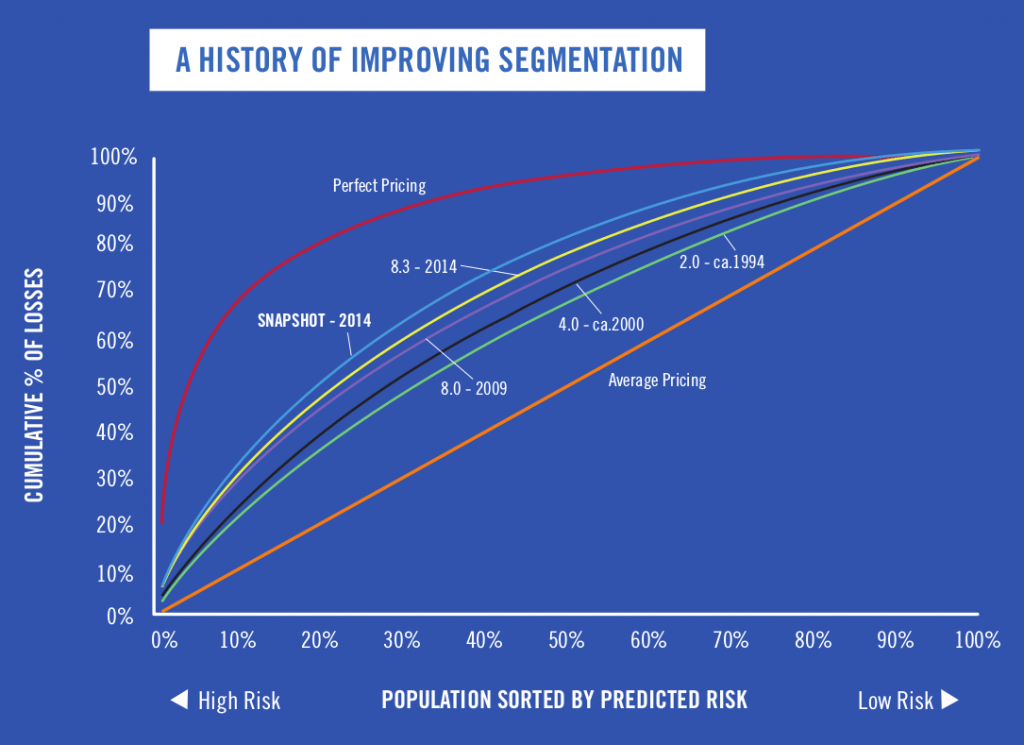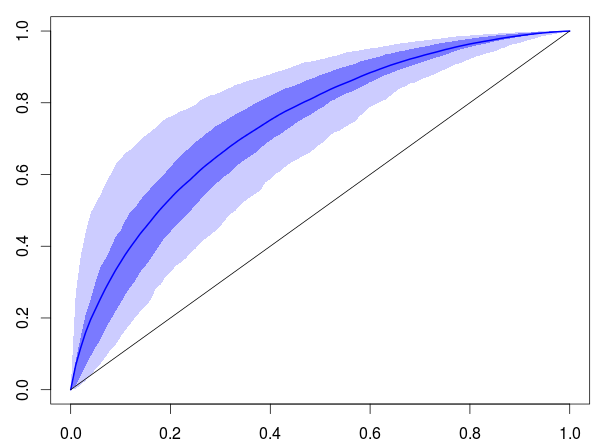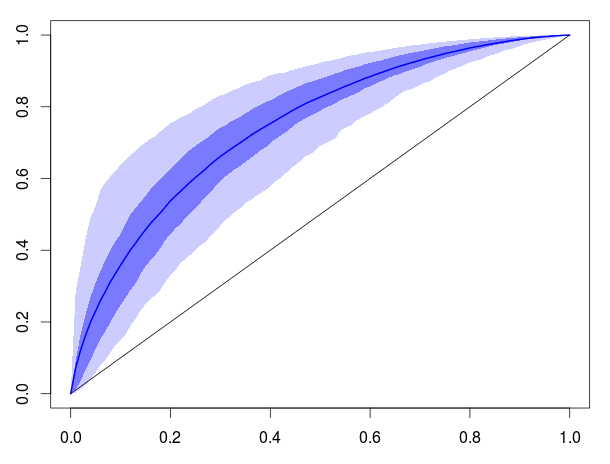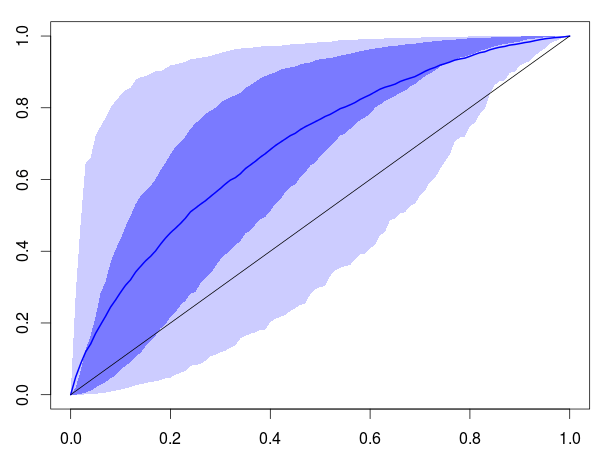[This article was first published on Freakonometrics » R-english, and kindly contributed to R-bloggers]. (You can report issue about the content on this page here)
Want to share your content on R-bloggers? click here if you have a blog, or here if you don't.

A few month ago, I did mention a graph, of some so-called Lorenz curves to compare regression models, see e.g. Progressive’s slides (thanks Guillaume for the reference)The idea is simple. Consider some model for the pure premium (in insurance, it is the quantity that we like to model), i.e. the conditional expected valeur

On some dataset, we have our predictions, as well as observed quantities, . The curve are obtained simply :

• sort the observations so that

• based on that ordering (from high risks to low risks, based on our predictions), we plot Lorenz curve

That looks nice, and as mentioned in my previous post, I have two issues regarding that graph. The first one is the ‘upper-bound’ : I do not understand this “perfect model”. The second one is the (pseudo) ‘lower-bound’, which is the “average model”. At least, for the later, I understand how to get it, but I do not understand what it means if we are (sometimes) below that line.

In order to understand a bit more, let us consider the following example.

• we have some covariates
• given those covariates, we have parameters , with some random effect
• given those parameters, we have observations

Let us generate such a dataset,

n = 10
s = 1
tau = 1
X = rnorm(n)
Theta = -1+X+rnorm(n)*tau
Y = exp(Theta+rnorm(n)*s)
base = data.frame(X,Theta,S,m)

and compare different strategies to get Lorenz curves :

• the random case : observations are ordered randomly. That simply means that predictions are identical
   Ys = rev(base[sample(1:n),"Y"])
L  = c(0,cumsum(Ys))/sum(Ys)
• the partial-information model : we fit a model using our covariates, and we sort observations base on that model
   reg = lm(log(Y)~X,data=base)
base$m = predict(reg) Ys = rev(base[order(base$m),"Y"])
L  = c(0,cumsum(Ys))/sum(Ys)
• a second partial-information model : assume that we know that the model should be a function increasing with the covariate. In that case, we can directly use it to sort our sample
   Ys = rev(base[order(base$X),"Y"]) L = c(0,cumsum(Ys))/sum(Ys) • the perfect model : here we observe . That’s clearly, here, the best we can do (we cannot observe the noise)  Ys = rev(base[order(base$Theta),"Y"])
L  = c(0,cumsum(Ys))/sum(Ys)

The empirical curve will be function of the number of observvations, , the variance of the random effect (that cannot be observed) , and the variance of the noise . In order to visualize what’s going on, let us generate several samples (say 1,000), and plot some confidence intervals (90% and 50%), as well as the average curve, obtained on those simulated samples. For instance, in the random case, use

L1=function(n=10,s=1,ns=1e3,tau=.2){
CY=matrix(NA,ns,n+1)
for(i in 1:ns){
X=rnorm(n)
Theta=-1+X+rnorm(n)/5
S=exp(Theta+rnorm(n)*s)
m=exp(Theta+.5*var(log(S)))
base=data.frame(X,Theta,S,m)
Y=rev(base[sample(1:n),"S"])
CY[i,]=c(0,cumsum(Y))/sum(Y)
}
Qinf1=apply(CY,2,function(x) quantile(x,.05))
Qsup1=apply(CY,2,function(x) quantile(x,.95))
Qinf2=apply(CY,2,function(x) quantile(x,.25))
Qsup2=apply(CY,2,function(x) quantile(x,.75))
Qm=apply(CY,2,mean)
return(data.frame(inf10=Qinf1,inf25=Qinf2,moy=Qm,sup75=Qsup2,sup90=Qsup1))
}

Then use

viz=function(B){
n=nrow(B)-1
u=(0:n)/n
plot(0:1,0:1,col="white",xlab="",ylab="")
polygon(c(u,rev(u)),c(B$inf10,rev(B$sup90)),col=rgb(0,0,1,.2),border=NA)
polygon(c(u,rev(u)),c(B$inf25,rev(B$sup75)),col=rgb(0,0,1,.4),border=NA)
lines(u,B$moy,col="blue",lwd=2) segments(0,0,1,1)} e.g. viz(L1(s=1,n=100,tau=1))Which is what we were expecting: we are around the diagonal, and on average, we have the diagonal. If we consider now the partial information model, L2=function(n=10,s=1,ns=1e3,tau=.2){ CY=matrix(NA,ns,n+1) for(i in 1:ns){ X=rnorm(n) Theta=-1+X+rnorm(n)*tau S=exp(Theta+rnorm(n)*s) m=exp(Theta+.5*var(log(S))) base=data.frame(X,Theta,S,m) Y=rev(base[order(base$X),"S"])
CY[i,]=c(0,cumsum(Y))/sum(Y)
}
Qinf1=apply(CY,2,function(x) quantile(x,.05))
Qsup1=apply(CY,2,function(x) quantile(x,.95))
Qinf2=apply(CY,2,function(x) quantile(x,.25))
Qsup2=apply(CY,2,function(x) quantile(x,.75))
Qm=apply(CY,2,mean)
return(data.frame(inf10=Qinf1,inf25=Qinf2,moy=Qm,sup75=Qsup2,sup90=Qsup1))
}

viz(L2(s=1,n=100,tau=1))We have here a curve above the first diagonal, as on the Figure mentioned in the introduction of this post. Note that if we use directly the covariate to sort, instead of  model, we have a rather similar graph,

L2b=function(n=10,s=1,ns=1e3,tau=.2){
CY=matrix(NA,ns,n+1)
for(i in 1:ns){
X=rnorm(n)
Theta=-1+X+rnorm(n)*tau
S=exp(Theta+rnorm(n)*s)
m=exp(Theta+.5*var(log(S)))
base=data.frame(X,Theta,S,m)
reg=lm(log(S)~X,data=base)
base$m=predict(reg) Y=rev(base[order(base$m),"S"])
CY[i,]=c(0,cumsum(Y))/sum(Y)
}
Qinf1=apply(CY,2,function(x) quantile(x,.05))
Qsup1=apply(CY,2,function(x) quantile(x,.95))
Qinf2=apply(CY,2,function(x) quantile(x,.25))
Qsup2=apply(CY,2,function(x) quantile(x,.75))
Qm=apply(CY,2,mean)
return(data.frame(inf10=Qinf1,inf25=Qinf2,moy=Qm,sup75=Qsup2,sup90=Qsup1))
}

viz(L2b(s=1,n=100,tau=1))And finally, our perfect model would be

L3=function(n=10,s=1,ns=1e3,tau=.2){
CY=matrix(NA,ns,n+1)
for(i in 1:ns){
X=rnorm(n)
Theta=-1+X+rnorm(n)*tau
S=exp(Theta+rnorm(n)*s)
m=exp(Theta+.5*var(log(S)))
base=data.frame(X,Theta,S,m)
Y=rev(base[order(base\$m),"S"])
CY[i,]=c(0,cumsum(Y))/sum(Y)
}
Qinf1=apply(CY,2,function(x) quantile(x,.05))
Qsup1=apply(CY,2,function(x) quantile(x,.95))
Qinf2=apply(CY,2,function(x) quantile(x,.25))
Qsup2=apply(CY,2,function(x) quantile(x,.75))
Qm=apply(CY,2,mean)
return(data.frame(inf10=Qinf1,inf25=Qinf2,moy=Qm,sup75=Qsup2,sup90=Qsup1))
}

viz(L3(s=1,n=100,tau=1))The curve is here in the upper left corner, again, as stated in the introductionary Figure.

But if we start playing with those functions, we can get weird results, e.g.

viz(L3(s=1,n=100,tau=1))Here, we have a large variability for the noise . This might happen in insurance when dealing with liabilty claims, with large variance, and heavy tails. In that case, observe that the perfect model can even be below the diagonal. Which makes me more and more suspicious regarding the use of such graphical tools in insurance, to assess if a model a good, or not. Here, the perfect model might seem worst than a random one (where an identical value for ‘s is considered, even if the true values of ‘s are given).

To leave a comment for the author, please follow the link and comment on their blog: Freakonometrics » R-english.

R-bloggers.com offers daily e-mail updates about R news and tutorials about learning R and many other topics. Click here if you're looking to post or find an R/data-science job.
Want to share your content on R-bloggers? click here if you have a blog, or here if you don't.

# Never miss an update! Subscribe to R-bloggers to receive e-mails with the latest R posts.(You will not see this message again.)

Click here to close (This popup will not appear again)